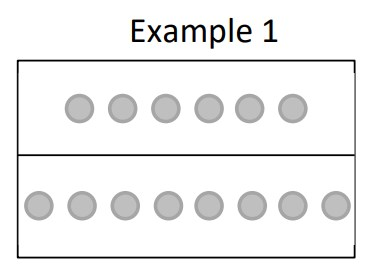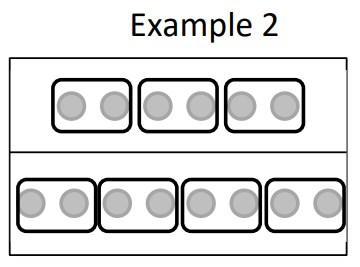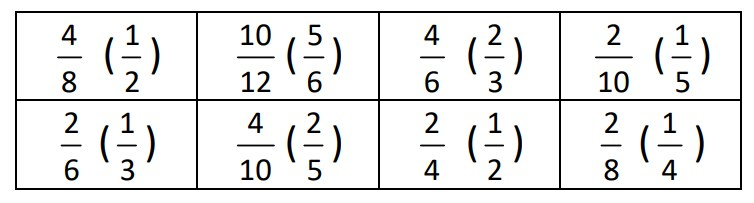# 5th Grade, Activity 171: Use Equivalent Fractions as a Strategy to Add and Subtract Fractions

Add and subtract fractions with unlike denominators (including mixed numbers) by replacing given fractions with equivalent fractions in such a way as to produce an equivalent sum or difference of fractions with like denominators.

For example, 2/3 + 5/4 = 8/12 + 15/12 = 23/12. (In general, a/b + c/d = (ad + bc)/bd.)

### Simplifying Fractions With Models

• Group: Pairs/Alone
• Materials: 8.5 x 11 sheet of paper, two color counters, paper and pencil, Simplifying Fractions with Models sheet, black markers

Issue 40 two-color counters to pairs, an 8.5 x 11 sheet of paper, and a black marker to each student. Instruct students to fold the paper in half, lengthwise. Have students trace over the folded crease with a black marker. Write the fraction 6⁄8 on the board. Instruct students to use the counters to model 6⁄8 by showing 6 counters above the line to represent the numerator and 8 counters below the line to represent the denominator as shown in Example 1 below.Place the counters in the numerator and denominator in largest possible equal-sized groups, as shown in Example 2 below. Emphasize that students must show the same number of equal groups for the numerator and denominator. The result shows that there are three groups of 3 in the numerator and four groups of 3 in the denominator. The simplified fraction is 34.Have students model how to simplify the fractions below with the counters.Issue the Simplifying Fractions With Models sheet to each student for them to complete independently. Ask questions relating to the greatest common factor of two numbers.